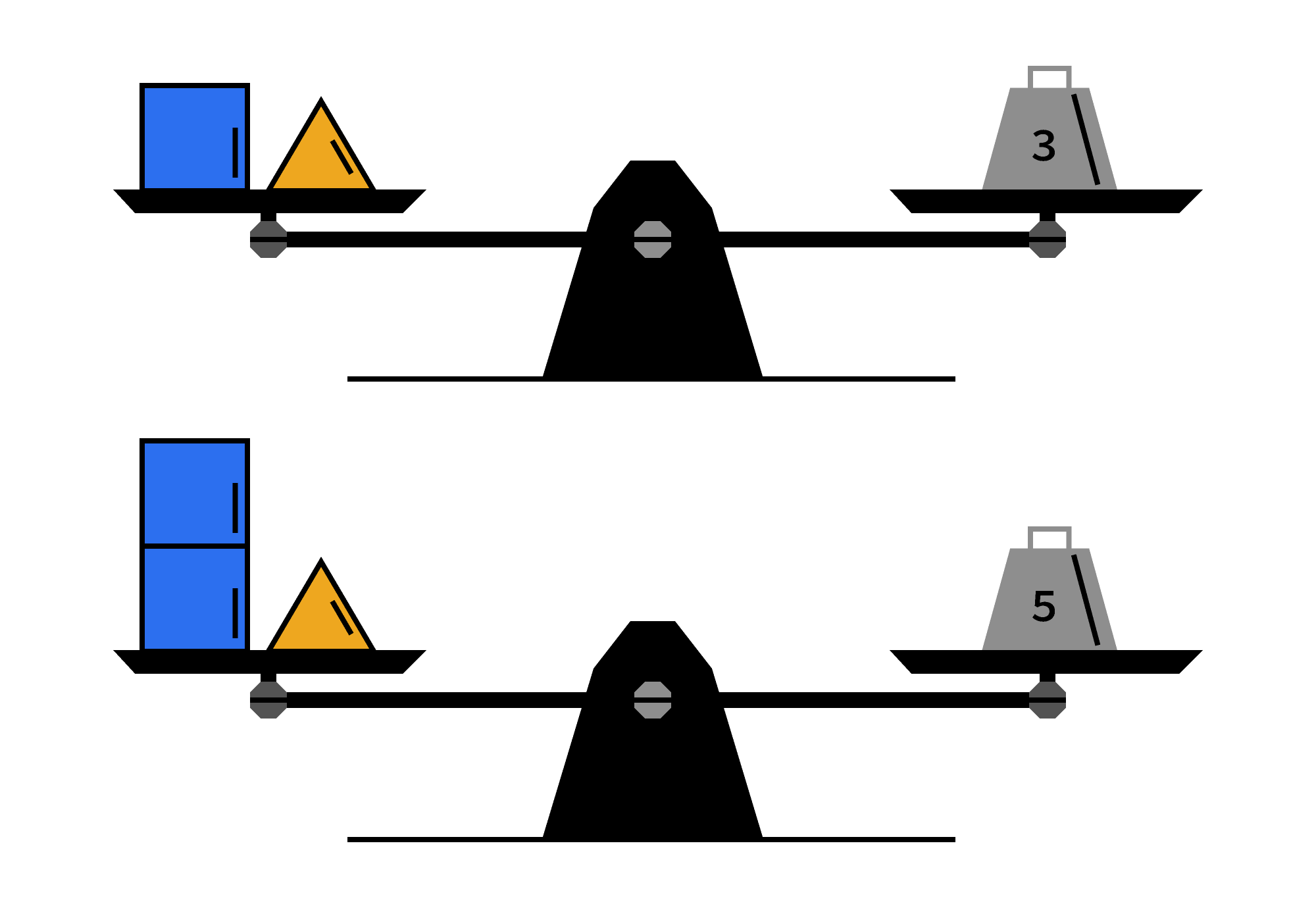## Off Balance

Given the two balance scales, we might ask how much the yellow triangle weighs:Or perhaps, we might want to know the value of $t$ in the equations \begin{aligned} s + t &= 3 \\ 2s + t &= 5. \end{aligned} In either case, we can use the same approach: the elimination method.